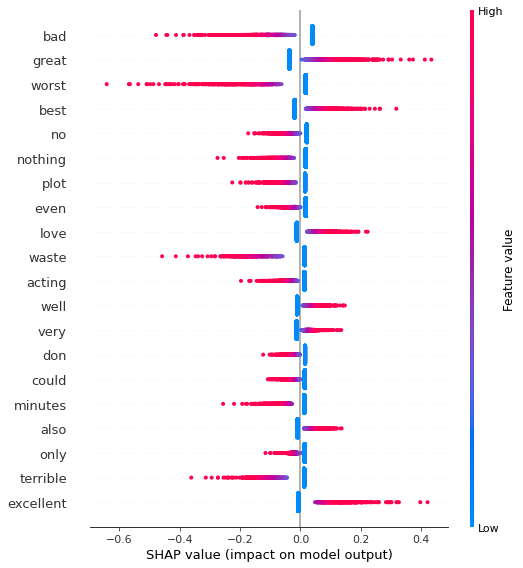In :
!pip install shap

Requirement already satisfied: shap in /usr/local/lib/python3.6/dist-packages (0.37.0)
Requirement already satisfied: numba in /usr/local/lib/python3.6/dist-packages (from shap) (0.48.0)
Requirement already satisfied: tqdm>4.25.0 in /usr/local/lib/python3.6/dist-packages (from shap) (4.41.1)
Requirement already satisfied: numpy in /usr/local/lib/python3.6/dist-packages (from shap) (1.18.5)
Requirement already satisfied: slicer==0.0.3 in /usr/local/lib/python3.6/dist-packages (from shap) (0.0.3)
Requirement already satisfied: pandas in /usr/local/lib/python3.6/dist-packages (from shap) (1.1.4)
Requirement already satisfied: scikit-learn in /usr/local/lib/python3.6/dist-packages (from shap) (0.22.2.post1)
Requirement already satisfied: scipy in /usr/local/lib/python3.6/dist-packages (from shap) (1.4.1)
Requirement already satisfied: llvmlite<0.32.0,>=0.31.0dev0 in /usr/local/lib/python3.6/dist-packages (from numba->shap) (0.31.0)
Requirement already satisfied: setuptools in /usr/local/lib/python3.6/dist-packages (from numba->shap) (50.3.2)
Requirement already satisfied: python-dateutil>=2.7.3 in /usr/local/lib/python3.6/dist-packages (from pandas->shap) (2.8.1)
Requirement already satisfied: pytz>=2017.2 in /usr/local/lib/python3.6/dist-packages (from pandas->shap) (2018.9)
Requirement already satisfied: joblib>=0.11 in /usr/local/lib/python3.6/dist-packages (from scikit-learn->shap) (0.17.0)
Requirement already satisfied: six>=1.5 in /usr/local/lib/python3.6/dist-packages (from python-dateutil>=2.7.3->pandas->shap) (1.15.0)

In :
from sklearn.feature_extraction.text import TfidfVectorizer
from sklearn.model_selection import train_test_split
from sklearn.linear_model import LogisticRegression

import numpy as np
import shap

shap.initjs()In :
corpus, y = shap.datasets.imdb()
corpus_train, corpus_test, y_train, y_test = train_test_split(corpus, y, test_size=.2, random_state=7)

vectorizer = TfidfVectorizer(binary=True)
X_train = vectorizer.fit_transform(corpus_train)
X_test = vectorizer.transform(corpus_test)

In :
model = LogisticRegression(C = .1)
model.fit(X_train, y_train)

Out:
LogisticRegression(C=0.1, class_weight=None, dual=False, fit_intercept=True,
intercept_scaling=1, l1_ratio=None, max_iter=100,
multi_class='auto', n_jobs=None, penalty='l2',
random_state=None, solver='lbfgs', tol=0.0001, verbose=0,
warm_start=False)
In :
explainer = shap.LinearExplainer(model, X_train, feature_dependence='independent')
shap_values = explainer.shap_values(X_test)
# we need to pass a dense version for the plotting functions
X_test_array = X_test.toarray()

The option feature_dependence has been renamed to feature_perturbation!
The option feature_perturbation="independent" is has been renamed to feature_perturbation="interventional"!

In :
shap.summary_plot(shap_values, X_test_array, feature_names=vectorizer.get_feature_names())In :
ind = 10

print("Positive" if y_test[ind] else "Negative", "Review:")
print(corpus_test[ind])

shap.initjs()
shap.force_plot(
explainer.expected_value, shap_values[ind,:], X_test_array[ind,:],
feature_names=vectorizer.get_feature_names()
)

Positive Review:
I would never have thought I would almost cry viewing one minute excerpted from a 1920 black and white movie without sound. Thanks to Martin Scorsese I did (the movie was from F. Borzage). You will start to understand (if it's not already the case), what makes a good movie.Out:
Visualization omitted, Javascript library not loaded!
Have you run initjs() in this notebook? If this notebook was from another user you must also trust this notebook (File -> Trust notebook). If you are viewing this notebook on github the Javascript has been stripped for security. If you are using JupyterLab this error is because a JupyterLab extension has not yet been written.